P R O F I L EG A L L E R YO R D E RC O N T A C T    I N F OE  X  P  E  R  I  M  E  N  T  A  L     W  O  R  K

"I am constantly a victim of paradigm thinking. Exploring new ideas can be rewarding and strengthens all areas of my work."

G A L L E R Y
F E A T U R E D   E X H I B I T
L A N D S C A P E
P E O P L E   &   L I F E S T Y L E
H U M A N   S H A P E   &   F O R M
S T I L L   L I F E
> E X P E R I M E N T A L   W O R K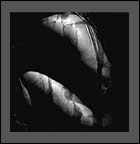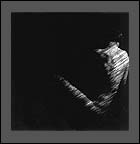t a r au n t i t l e d  1j o d i e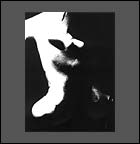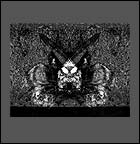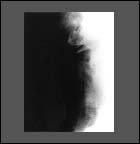u n t i t l e d  2d e m o np a i n t e d   o u t >  S E N D   T H I S   S I T E   T O   A   F R I E N DT O P© 2001 - 2005   R o b e r  t   J   W i l k i n s o n             P r i v a c y   P o l i c ys i t e   d e s i g n